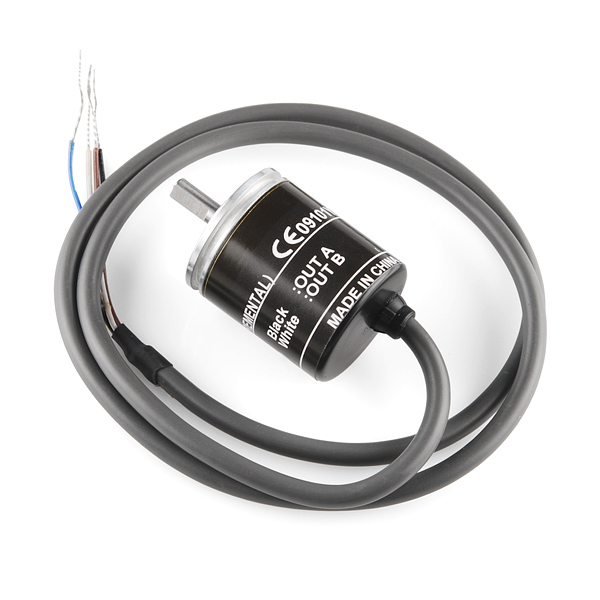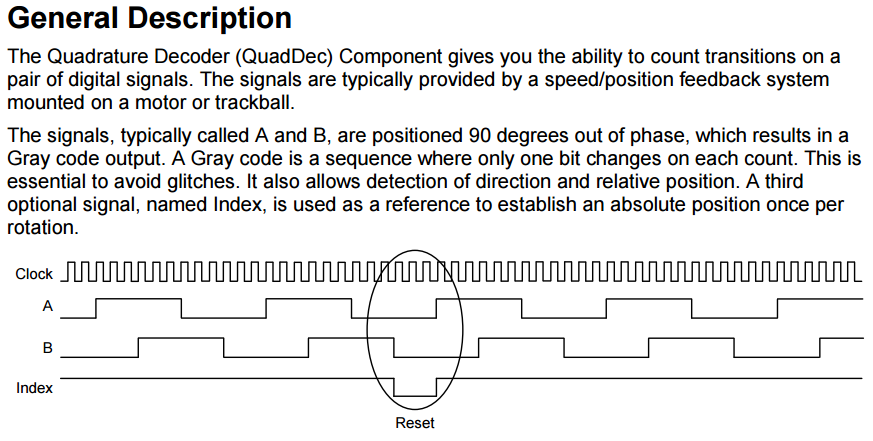## Physics Lab: The Rotary Encoder

When I started thinking about the G-Force machine I knew that I wanted to be able teach Newtons Laws using data collected from the machine.  That meant that I needed position, velocity and acceleration.  Since we all went to Physics class, we remember that you can calculate velocity from the change in position, and you can calculate acceleration from the change in velocity.

Given all of that, I decided to start with an E6A2-CW3C rotary quadrature encoder which I bought from Sparkfun.  This device sends out two trains of pulses based on the direction and speed of the rotor.  There is/will be a wheel attached to the rotor of this device that will run along the track.  As the car moves, it will turn the rotor and send out the train of pulses which can then be connected the PSoC TCPWM which is a block inside of the PSoC.   These pulses are encoded with a scheme known as “quadrature encoding”.The next picture is a snapshot from the Cypress Quadrature Decoder Component Datasheet which explains quadrature.The E6A2-CW3C quadrature decoder generates 200 pulses per rotation.  Assuming that the diameter of the wheel that I attach to the device is 10″, I will be able to measure a change of 10″/200 pulses = 0.05 inch.  More than enough.  In fact the PSoC Quadrature component is capable of counting rising and falling edges which yields a multiplier up to 4x or 0.0126″ (which is beyond ridiculous).  The one issue that is important with the design is that I would like the total number of pulses that the system generates as it moves down the track to be able to fit into a 16-bit number.  Given that the track is 120′ long, that means with a 10″ wheel I will get 120 feet*12 inches/foot / 10inches/turn = 144 turns * 200 pulses/turn = 28,800 pulses for the entire length of the track.  This is good as it is well less than 2^16=65536 which is the largest number that can fit into a 16-bit number.

In the next post Ill talk more about the Accelerometer(s).

Index Description
Physics Lab: An Introduction to G-Force Introduction
Physics Lab: The Rotary Encoder Measuring position